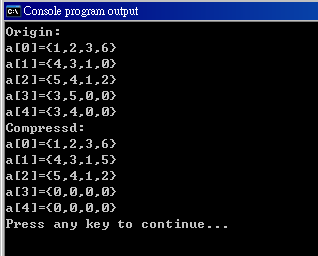#1

## 原陣列

a={{1,2,3,6},
{4,3,1},
{5,4,1,2},
{3,5},
{3,4}};

b={{1,2,3,6},
{4,3,1,5},
{5,4,1,2}};

34 可以跟 4315 合

weiclin iT邦高手 4 級 ‧ 2018-11-03 17:34:13 檢舉

### 5 個回答

4if``````#include <stdio.h>
#include <string.h>
#include <stdarg.h>
#include <stdint.h>

#define DATA_LENGTH 5

typedef struct{
int length; //儲存幾個數字
int buf;
unsigned mask;
} set;

void setDatas(set* p, int n, ...);
void showSetArray(int n,set **arr);

int main()
{
set database[DATA_LENGTH];
set *arr[DATA_LENGTH];
int i,j,n=DATA_LENGTH,k,count;
unsigned mask;

//因為最多只有 1024 種組合，可以利用查表加速
//下面這個陣列可以查 mask 位元不為 0 的數量是否 <= 4
//即(bit_count[mask/32]&1<<mask%32)若為 true 代表可合併
uint32_t bit_count[]={
0x7fffffff,0x177fffff,0x177f7fff,0x01571fff,
0x177f7fff,0x77d77f7f,0x01d73fff,0x0061ffdf,
0x77ff7fff,0x77df577f,0x0177ff7f,0x76c7639f,
0x0117177f,0x76c76bd7,0x76c76bbf,0xb86d0d85,
0x177f7fff,0x015717ff,0x0117177f,0x004119f7,
0x0177ffff,0x0061ff1f,0x0061ffdf,0x76c6ca21,
0xcfd77fff,0xffffffff,0x76c76bbf,0x76c66cad,
0x004119f7,0x0060ff95,0x00401a4b,0x004019f0
};

//測試資料
setDatas(database+0,4,1,2,3,6);
setDatas(database+1,3,4,3,1);
setDatas(database+2,4,5,4,1,2);
setDatas(database+3,2,3,5);
setDatas(database+4,2,3,4);

//用指標指向每一列，方便操作
//(刪除、移動時減少記憶體的讀寫量)
for(i=0;i<DATA_LENGTH;++i)
arr[i]=database+i;

//顯示測試資料(初始狀態)
printf("initial:\n");
showSetArray(n,arr);
printf("-------------\n\n");

//開始壓縮
for(i=1;i<n;++i)
{
//子集合處理
k=0;
for(j=0;j<i;++j)
{
//利用位元運算判斷誰是誰的子集合
mask=arr[i]->mask|arr[j]->mask;
if(mask==arr[j]->mask)
{// i 為 j 的子集, 刪除 i
memmove(arr+i,arr+i+1,(--n-i)*sizeof(*arr));
--i;
k=1;
break;
}
if(mask==arr[i]->mask)
{// j 為 i 的子集, 用 i 取代 j
arr[j]=arr[i];
memmove(arr+i,arr+i+1,(--n-i)*sizeof(*arr));
--i;
k=1;
break;
}
}
if(k)
continue;

//如果不存在子集合關係，嘗試合併集合
for(j=0;j<i;++j)
{
if(arr[j]->length>=4)
continue;
//聯集後，計算有幾個不為0的位元，即為合併後所需空間
mask=arr[i]->mask|arr[j]->mask;

/*
for(count=k=0;k<10;++k)
if(1<<k&mask)
++count;

if(count>4)//合併後會超過
continue;
*/

//查表加速，等同於上面註解掉的程式碼
if(~bit_count[mask/32]>>mask%32&1) //合併後會超過
continue;

//合併後不會超過的話執行以下程序合併
for(k=0;k<arr[i]->length;++k)
{
mask=1<<arr[i]->buf[k];
if(!(mask&arr[j]->mask))
{
arr[j]->mask|=mask;
arr[j]->buf[arr[j]->length++]=arr[i]->buf[k];
}
}
memmove(arr+i,arr+i+1,(--n-i)*sizeof(*arr));
--i;
break;
}
}
//顯示結果
printf("result:\n");
showSetArray(n,arr);
printf("-------------\n\n");
return 0;
}

void setDatas(set* p, int n, ...)
{
int x;
p->length=0;
va_list args;
va_start(args,n);
p->mask=0;
while(n--)
{
x=va_arg(args,int);
p->buf[p->length++]=x;
p->mask|=1<<x;
}
va_end(args);
}
void showSetArray(int n,set **arr)
{
int i,j;
for(i=0;i<n;++i)
{
printf("%02X ",arr[i]->mask);
for(j=0;j<arr[i]->length;++j)
printf(" %d%c",arr[i]->buf[j],arr[i]->length-1==j?'\n':',');
}
}
``````

weiclin iT邦高手 4 級 ‧ 2018-11-03 23:41:37 檢舉

weiclin iT邦高手 4 級 ‧ 2018-11-04 12:04:07 檢舉``````for(count=k=0;k<10;++k)
if(1<<k&mask)
++count;

if(count>4)//合併後會超過
continue;
````````````int bit_array,i;
for(i=0;i<1024;++i)
if(i 不超過 4 個 bit 是 1)
bit_array[i]=1;
else
bit_array[i]=0;
``````

(每個 uint32_t 有 32 個位元，共有 32 個 uint32_t，合計 1024 bit)

weiclin iT邦高手 4 級 ‧ 2018-11-04 18:10:28 檢舉1

``````column = 4
for (i = 1 ~ (Array.size - 1)){
for ( j = 0 ~ (i - 1)){
if ((num of elements that not in Array[j]) > (column-Array[j].size)){
j++
if (j = i) i++
}
else {
add (elements that not in Array[j]) to Array[j]
remove Array[i]
Array.size - 1
break
}
}
}
``````

(有錯的話請糾正 > <)

0

1

Pelles C (http://www.pellesc.de/)

``````#include <stdio.h>
int InList(char a, char x)
{
int k;
for (k = 0; k < 4; k++)
{
if (a == x[k])
return 1;
}
return 0;
}
int GetCount(char x)
{
int k;
for (k = 0; k < 4; k++)
{
if (x[k] == 0)
return k;
}
return 4;
}
int GetSpace(char x)
{
return (4 - GetCount(x));
}
void Compress(char t, char x)
{
int i, k;
for (i =0; i < 4; i++)
{
if (t[i] == 0)
break;
if (InList(t[i], x))
t[i] = 0;
else
{
x[GetCount(x)] = t[i];
for (k = i; ++k < 4;)
{
t[k-1] = t[k];
if (t[k] == 0)
break;
}
i--;
}
}
}
void ShowArray(char a)
{
int i, j;
for (i = 0; i < 5; i++)
{
printf("a[%d]=", i);
for (j = 0; j < 4; j++)
printf("%c%d", (j==0)?'{':',', a[i][j]);
printf("}\n");
}
}
int main(int argc, char *argv[])
{
char a={
{1,2,3,6},
{4,3,1,0},
{5,4,1,2},
{3,5,0,0},
{3,4,0,0}
};

int i, j, k;
int count, need;

printf("Origin:\n");
ShowArray(a);

for (i = 1; i < 5; i++)
{
for (j = 0; j < i; j++)
{
k = 0;
need = 0;
count = 0;
for (k = 0; k < 4; k++)
{
if (a[i][k] == 0)
break;
if (InList(a[i][k], a[j]))
count++;
else
need++;
}
if (need > 0)
{
if (need <= GetSpace(a[j]))
{
Compress(a[i], a[j]);
}
}
else if (count == GetCount(a[i]))
{
while (k > 0)
a[i][--k] =0;
}
}
}

printf("Compressd:\n");
ShowArray(a);

return 0;
}

``````0

arr1即是答案

``````#include<stdio.h>
#include<stdlib.h>

int main()
{
char temp = {'\0'};
int arr1;
int arr2 = {
{ 1,2,3,6 },
{ 4,3,1 },
{ 5,4,1,2 },
{ 3,5 },
{ 3,4 }
};

for (int i = 0; i < 5; i++)
{
for (int j = 0; j < 4; j++)
{
if(arr2[i][j]==0)
sprintf_s(temp, "%s%c", temp, 'x');
else
sprintf_s(temp, "%s%d", temp, arr2[i][j]);
}
}

int row = 0, column = 0;
for (int count = 0; count < 100; count++)
{
if (temp[count] != 'x')
{
if (column == 4)
{
column = 0; row++;
}
if (row == 5)
break;

arr1[row][column] = temp[count] - 48; //char to int
column++;
}
}

system("pause");
return 0;
}
``````